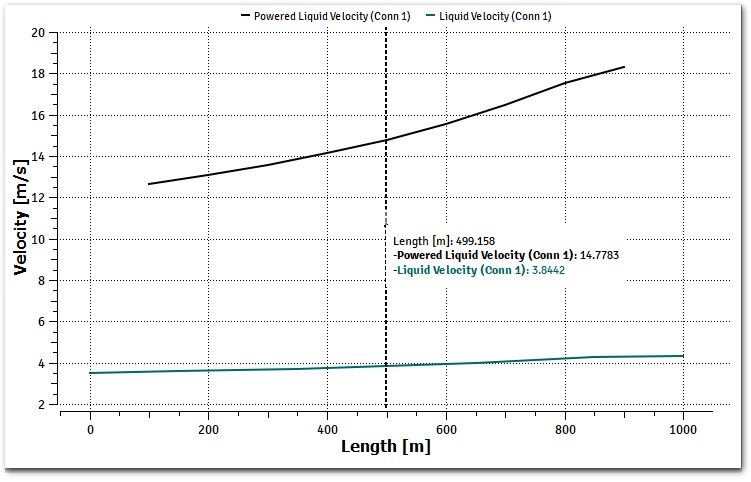# Plugin by Example#

In this section, we will continue to customize the plugin created from the previous section, this plugin will calculate the oil velocity to the power of a given exponent provided by the user from the user interface.

The following equation describes the plugin:

$var = U_{\text{oil}}^{n}$

Where

$$U_{\text{oil}}$$:

Oil Velocity

$$n$$:

Exponent, input from user interface

$$var$$:

Plugin calculated value, that will be shown on the output.

For this, we will need to:

1. Create a simple input, on the user interface.

2. Add a secondary variable, to keep track of the value.

3. Retrieve the input data on the HOOK_INITIALIZE.

4. Save the input data on a plugin internal data.

5. Get the oil velocity from the solver, during run-time.

6. Export the value into the created/registered plugin secondary variable.

## Application customization#

ALFAsim-SDK allows plugins to customize the user interface of ALFAsim by adding custom models, the image below shows the locations where a custom model can be inserted using the hook alfasim_get_data_model_type().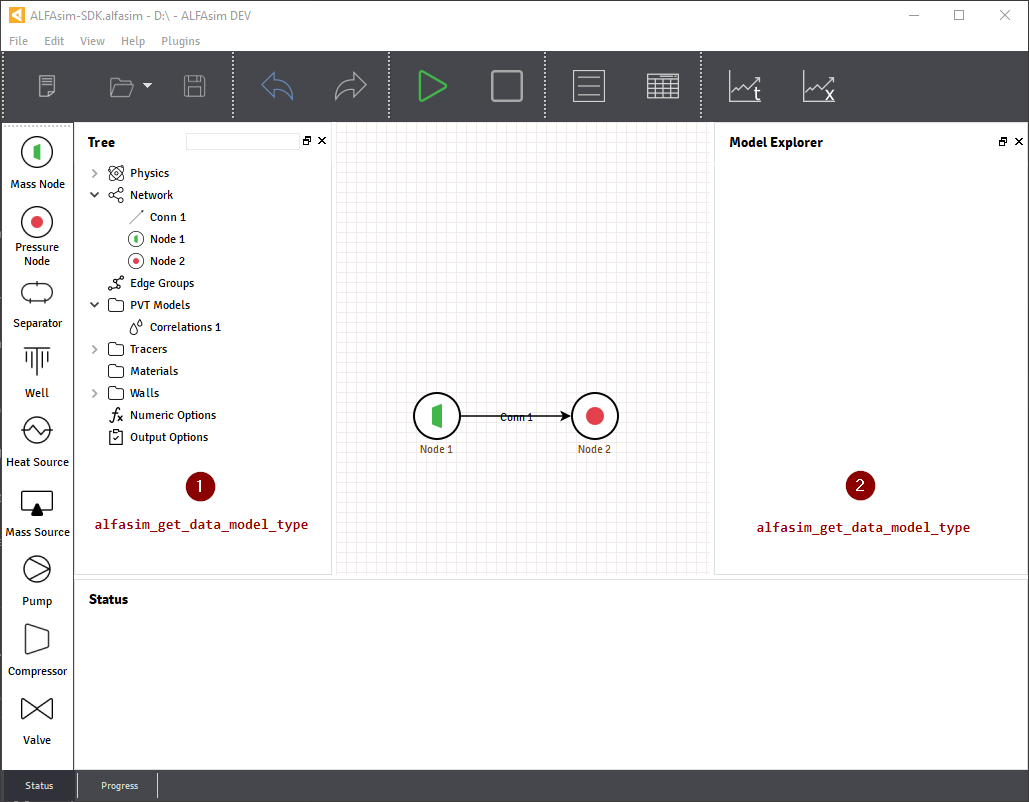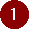illustrates the section where the models container_model() or data_model() will be placed.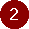illustrates the section where the inputs fields will be placed.

For this example we will use a data_model() entry over the Tree, using a Quantity field to get exponent value from the user.

The hook alfasim_get_data_model_type() needs to be implemented on the myplugin.py, located on myplugin/src/python folder.

Implementation of myplugin.py

import alfasim_sdk
from alfasim_sdk import data_model
from alfasim_sdk import Quantity

@data_model(caption='My plugin model', icon='')
class MyPluginModel:
exponent = Quantity(value=1, unit='-', caption='Exponent value')

@alfasim_sdk.hookimpl
def alfasim_get_data_model_type():
return [MyPluginModel]


Notice that only models that are returned by the hook alfasim_get_data_model_type() will be included on the user interface of ALFAsim.

The image below illustrates the application with the output from the snippet above.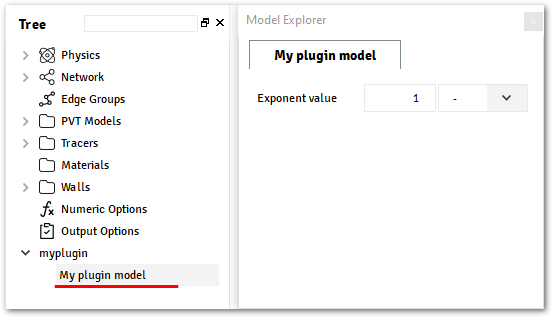For more details about all input fields available, check the section Types. And for more detail about the available models, check the section Models.

## Solver Configuration#

ALFAsim-SDK provides hooks to customize the settings of the application that configures the solver internally, some of these configurations are:

• Creation/Registration of new secondary variables

• Creation of new phases/fields/layers.

• Update of default phases and layers from the application.

For this example, a new SecondaryVariable() will be created, to track the oil velocity to the power of a custom value provided from the user.

A Secondary Variable is a variable that can be calculated along the Network. Also, if configured as external, this variable will be set an Output, and will be available within the Trends and Profiles plots.

To create these variables, the hook alfasim_get_additional_variables() must be implemented in the myplugin.py file.

Implementation of myplugin.py

@alfasim_sdk.hookimpl
import alfasim_sdk
from alfasim_sdk import SecondaryVariable
from alfasim_sdk import Visibility
from alfasim_sdk import Location
from alfasim_sdk import Scope

return [
SecondaryVariable(
name='U_oil_n',
caption='Powered Oil Velocity',
unit='-',
visibility=Visibility.Output,
location=Location.Center,
multifield_scope=Scope.Global,
checked_on_gui_default=True,
),
]


The image below illustrates the application with the output from the snippet above. To access that window, first select the desired structure then go to Output Options at the left side tree and add/edit a trend.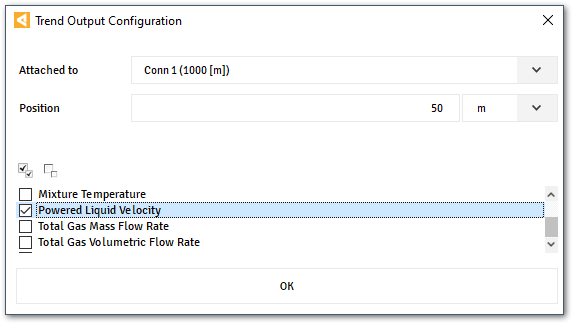For more details about SecondaryVariable, check the section Secondary Variables.

## Hooks for Solver#

ALFAsim-SDK provides hooks that can customize the Solver behavior, this customization are implemented in C/C++ and can make use of the ALFAsim-SDK C/C++ API in order to fetch information from the application.

At this point, we are going to implement the Solver Hooks that updates the secondary variable declared from myplugin.py file and retrieve the Oil Velocity from the ALFAsim’s Solver.

First, we need to implement two mandatory hooks, the HOOK_INITIALIZE and the HOOK_FINALIZE

With them it is possible to initialize any custom data (to store any important information) for internal use. Also it is needed to load and unload the ALFAsim-SDK API, in which will allows the plugin to use the API in any implemented hook.

Implementation of myplugin.cpp

#include "hook_specs.h"
#include <alfasim_sdk_api/alfasim_sdk.h>
#include <iostream>

ALFAsimSDK_API alfasim_sdk_api;

struct MyPluginModel {
double exponential = 0.0;
};

HOOK_INITIALIZE(ctx)
{
alfasim_sdk_open(&alfasim_sdk_api);

int errcode = -1;
double n = 0.0;

errcode = alfasim_sdk_api.get_plugin_input_data_quantity(
ctx,
&n,
get_plugin_id(),
(const char*) "MyPluginModel.exponent");
if (errcode != 0) {
std::cout << "input_data_quantity error=" << errcode << "\n";
return errcode;
}

// MyPluginModel is a class or struct defined by plugin
auto* model = new MyPluginModel();
model->exponential = n;
errcode = alfasim_sdk_api.set_plugin_data(
ctx,
get_plugin_id(),
(void*) model,
);
}

return OK;
}

HOOK_FINALIZE(ctx)
{

auto errcode = -1;
MyPluginModel* model = nullptr;
errcode = alfasim_sdk_api.get_plugin_data(ctx, (void**) (&model), get_plugin_id(), thread_id);
delete model;
}
alfasim_sdk_close(&alfasim_sdk_api);

return OK;
}


Then, since the plugin wants to calculate its own secondary variable, the HOOK_UPDATE_PLUGINS_SECONDARY_VARIABLES must be implemented. As can be seen in the example below, to retrieve the velocity of the continuous oil field it is necessary to use the get_simulation_array() API function.

HOOK_UPDATE_PLUGINS_SECONDARY_VARIABLES(ctx)
{
int errcode = -1;

// Get Oil Field ID
int oil_field_id = -1;
errcode = alfasim_sdk_api.get_field_id(
ctx,
&oil_field_id,
"oil"
)
if (errcode != 0) {
std::cout << "get_oil_id error = " << errcode << "\n";
return errcode;
}

// Get Oil Field Velocity
int n_faces = -1;
double* U_oil = nullptr;
errcode = alfasim_sdk_api.get_simulation_array(
ctx,
&U_oil,
(char*) "U",
VariableScope {
GridScope::CENTER,
MultiFieldDescriptionScope::FIELD,
TimestepScope::CURRENT
},
oil_field_id,
&n_faces);
if (errcode != 0) {
std::cout << "get_simulation_array error = " << errcode << "\n";
return OK;
}

// Get Exponent input data
double n = 0.0;
{

MyPluginModel* model = nullptr;
errcode = alfasim_sdk_api.get_plugin_data(
ctx, (void**) (&model),
);
n = model->exponential;
}

// Get Plugin Secondary Variable
int size = -1;
double* U_oil_n_ptr = nullptr;
errcode = alfasim_sdk_api.get_plugin_variable(
ctx,
(void**) &U_oil_n_ptr,
"U_oil_n",
0, // Global Scope
TimestepScope::CURRENT,
&size);
if (errcode != 0) {
std::cout << "get_plugin_variable error = " << errcode << "\n";
return errcode;
}
// Calculating the 'U_oil' to power of 'n'
for (int i = 0; i < size; ++i) {
U_oil_n_ptr[i] = std::pow(U_oil[i], n);
};

return OK;
}


The image below illustrates the output from the solver, when running the plugin created in this section with the given network. That information can be seen by selecting the desired structure and opening the Trend plot window.